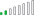cancel
Showing results for
Did you mean:Helper I

## Financial accounting - Sum account

Hey there,

We have a problem with figuring out how to calculate a total sum of our "sum accounts".

Right now we have a table with "sum accounts no." which contains values such as:

"11100.11199" which means that the accounts in that range is the reason for the total calculation..

Then we have a table with all the accounts that are within that range.

11100, 11101, 11102 etc.

Is there any "easy" way to calculate a total, without creating a new column with List.numbers and then do a sum with them all ?

1 ACCEPTED SOLUTIONCommunity Support

First split the "sum accounts no." column to 2 columns,then create a measure similarly as below:

``````Measure =
CALCULATE(SUM('Table (2)'[Value]),FILTER(ALL('Table (2)'),'Table (2)'[No.]>=MAX('Table'[sum accounts no - Copy.1])&&'Table (2)'[No.]<=MAX('Table'[sum accounts no - Copy.2])))``````

And you will see:For the related .pbix file,pls see attached.

Best Regards,
Kelly
5 REPLIES 5Community Support

First split the "sum accounts no." column to 2 columns,then create a measure similarly as below:

``````Measure =
CALCULATE(SUM('Table (2)'[Value]),FILTER(ALL('Table (2)'),'Table (2)'[No.]>=MAX('Table'[sum accounts no - Copy.1])&&'Table (2)'[No.]<=MAX('Table'[sum accounts no - Copy.2])))``````

And you will see:For the related .pbix file,pls see attached.

Best Regards,
KellyResolver II

As other said, you should split the column then get Account within the range, eventually creaing a bridge table. Here is a sample code for doing so:

``````// FinancialAccounting
let
Source = Table.FromRows(Json.Document(Binary.Decompress(Binary.FromText("i45WMjQ0NDDQA5KWlkqxOkA+iGdkAGGbGugZGQDZsQA=", BinaryEncoding.Base64), Compression.Deflate)), let _t = ((type nullable text) meta [Serialized.Text = true]) in type table [SumAccountsNo = _t]),
#"Changed Type" = Table.TransformColumnTypes(Source,{{"SumAccountsNo", type text}}),
#"Split Column by Delimiter" = Table.SplitColumn(#"Changed Type", "SumAccountsNo", Splitter.SplitTextByDelimiter(".", QuoteStyle.Csv), {"AccountNoLow", "AccountNoHigh"}),
#"Changed Type1" = Table.TransformColumnTypes(#"Split Column by Delimiter",{{"AccountNoLow", Int64.Type}, {"AccountNoHigh", Int64.Type}}),
#"Expanded Custom" = Table.ExpandListColumn(#"Added Custom", "Custom")
in
#"Expanded Custom"``````Super User

You should create a new columns and split these values to StartAccount and EndAccount

Based on those new columns you should create your SUM measures by filtering accounts

Proud to be a Super User!Super User

@JessVinsteen , as this a range, I do see an easy way out. Some calculation needs to be done.

Can you share sample data and sample output in table format?Helper I

Unfortunately Im not able to post any power bi files here, due to coporate rules...